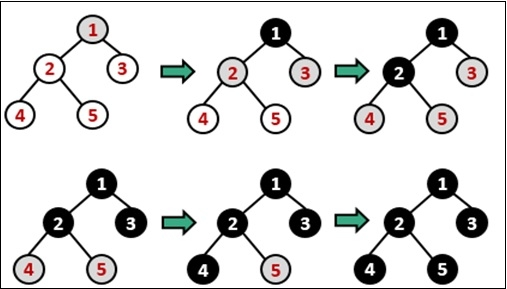# Breadth First Search (BFS) for a Graph

Data StructureGraph AlgorithmsAlgorithms

The Breadth First Search (BFS) traversal is an algorithm, which is used to visit all of the nodes of a given graph. In this traversal algorithm one node is selected and then all of the adjacent nodes are visited one by one. After completing all of the adjacent vertices, it moves further to check another vertex and checks its adjacent vertices again.To implement this algorithm, we need to use the Queue data structure. All the adjacent vertices are added into the queue when all adjacent vertices are completed, one item is removed from the queue and start traversing through that vertex again.

In Graph sometimes, we may get some cycles, so we will use an array to mark when a node is visited already or not.

## Input and Output

Input:
The Adjacency matrix of the graph.

A B C D E F
A 0 1 1 1 0 0
B 1 0 0 1 1 0
C 1 0 0 1 0 1
D 1 1 1 0 1 1
E 0 1 0 1 0 1
F 0 0 1 1 1 0

Output:
BFS Traversal: B A D E C F

## Algorithm

bfs(vertices, start)

Input − The list of vertices, and the start vertex.

Output − Traverse all of the nodes, if the graph is connected.

Begin
define an empty queue que
at first mark all nodes status as unvisited
add the start vertex into the que

while que is not empty, do
delete item from que and set to u
display the vertex u

for all vertices 1 adjacent with u, do
if vertices[i] is unvisited, then
mark vertices[i] as temporarily visited
mark
done

mark u as completely visited
done
End

## Example

#include<iostream>
#include<queue>
#define NODE 6
using namespace std;

typedef struct node {
int val;
int state;    //status
}node;

int graph[NODE][NODE] = {
{0, 1, 1, 1, 0, 0},
{1, 0, 0, 1, 1, 0},
{1, 0, 0, 1, 0, 1},
{1, 1, 1, 0, 1, 1},
{0, 1, 0, 1, 0, 1},
{0, 0, 1, 1, 1, 0}
};

void bfs(node *vert, node s) {
node u;
int i, j;
queue<node> que;

for(i = 0; i<NODE; i++) {
vert[i].state = 0;    //not visited
}

vert[s.val].state = 1;   //visited
que.push(s);            //insert starting node

while(!que.empty()) {
u = que.front();    //delete from queue and print
que.pop();
cout << char(u.val+'A') << " ";

for(i = 0; i<NODE; i++) {
if(graph[i][u.val]) {
//when the node is non-visited
if(vert[i].state == 0) {
vert[i].state = 1;
que.push(vert[i]);
}
}
}
u.state = 2;   //completed for node u
}
}

int main() {
node vertices[NODE];
node start;
char s;

for(int i = 0; i<NODE; i++) {
vertices[i].val = i;
}

s = 'B';   //starting vertex B
start.val = s-'A';
cout << "BFS Traversal: ";
bfs(vertices, start);
cout << endl;
}

## Output

BFS Traversal: B A D E C F